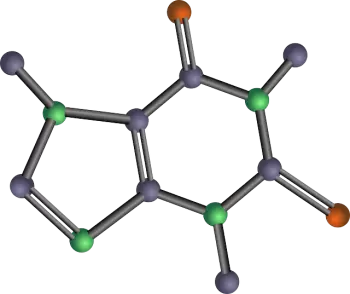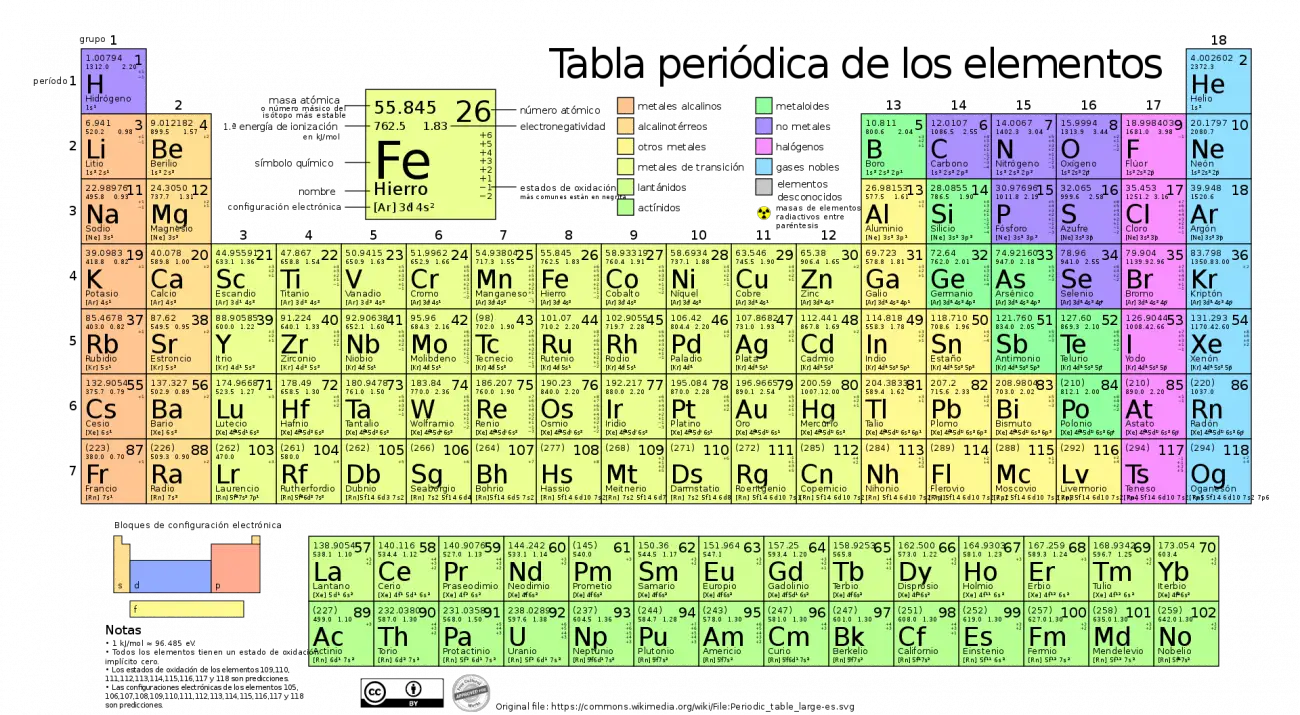# Molar Mass: Definition, Calculation, Use and ApplicationsMolar mass is a physical property used to describe the average mass of an atom or molecule of a substance in units of grams per mole (g/mol) and is represented by the letter "M."

In other words, molar mass indicates how many grams of a substance are in one mole of that substance. The mole is a unit of quantity of substance in the International System of Units (SI).

## Measurement Units

In the International System of Units (SI), the unit for measuring molar mass is kilogram per mole (kg/mol). However, when the molar mass is expressed in g/mol, its numerical value is the same with the relative molecular weight. For this reason, historically molar weight is generally expressed in grams per mole (g/mol).

Molar mass in formulas is usually indicated by a capital letter M.

Direct comparisons and measurements of the masses of atoms and molecules are made using mass spectrometry methods.

## Molar Mass Calculation

Calculating the molar mass of a compound is done by adding the masses of all the atoms in a molecule using the relative atomic masses.

Relative atomic masses are numbers that represent the mass of an atom relative to the mass of the carbon-12 atom, which is defined as 12 atomic mass units (amu).

For example, the relative atomic mass of hydrogen is approximately 1 amu, while the relative atomic mass of oxygen is approximately 16 amu.

### Complex MoleculesTo calculate the number of molar masses of complex molecules, it can be determined by adding the molar masses of their constituent elements. For example, the molar mass of water H 2 O is

M(H 2 O) = 2 M(H) + M(O) = 2.1 g/mol + 16 g/mol = 18.1 g/mol

### How to Calculate Molar Mass Step by Step?

Calculating the molar mass of a chemical substance can be done by following these steps:

1. Identify the chemical formula of the chemical compound.

2. Determine how many atoms of each element there are in the chemical formula.

3. Multiply the number of atoms of each element by its relative atomic mass.

4. Add the masses of all the atoms in the formula to obtain the molar mass.

### Practical Example

Let's look at a concrete example to calculate the molar mass of water (H₂O):

• The chemical formula of water is H₂O, which means it contains two hydrogen atoms (H) and one oxygen atom (O).

• The relative atomic mass of hydrogen is approximately 1 amu and that of oxygen is approximately 16 amu.

• To calculate the molar mass of water, we add the masses of the atoms: (2 * 1 amu) + (1 * 16 amu) = 2 amu + 16 amu = 18 amu.

Therefore, the molar mass of water is 18 g/mol.

## Examples

Below is a table with examples of some molar masses of different substances:

 substance Chemical formula Molar mass (g/mol) Description Hydrogen H₂ 2 Hydrogen is the lightest element, with a molar mass of 2 g/mol. Oxygen O₂ 32 Oxygen is essential for respiration and has a molar mass of 32 g/mol. Carbon dioxide CO₂ 44 CO₂ is a greenhouse gas with a molar mass of 44 g/mol. Ammonia NH₃ 17 Ammonia is used in the chemical industry and has a molar mass of 17 g/mol. Methane CH₄ 16 Methane is the main component of natural gas and has a molar mass of 16 g/mol. Sulfuric acid H₂SO₄ 98 Sulfuric acid is a widely used strong acid with a molar mass of 98 g/mol. Glucose C₆H₁₂O₆ 180 Glucose is a sugar found in foods and has a molar mass of 180 g/mol. Sodium Chloride (table salt) NaCl 58.5 Sodium chloride is common table salt and has a molar mass of 58.5 g/mol. Uranium OR 238.03 Uranium is an element used as fuel in nuclear reactors. Plutonium Pu 244 Plutonium is an element used in nuclear weapons and nuclear reactors. Heavy Water D₂O 20.03 Heavy water contains deuterium instead of hydrogen and is used in nuclear reactors. Enriched uranium U-235 It varies Enriched uranium contains a higher proportion of U-235 and is essential for nuclear fission.

## What Is Molar Mass Used For?

Molar mass is a fundamental property that has several implications and applications in chemistry and other scientific disciplines.

Some of its most notable applications include:

• Conversion between moles and grams: used to convert between the amount of substance in moles and the mass in grams of a substance.

• Determination of the composition of substances.

• Calculation of chemical reactions: It is essential for calculating the quantities of reactants and products in a chemical reaction, allowing for stoichiometry and experiment planning.

• Identification of unknown substances: Used in analytical techniques, such as mass spectroscopy, to identify unknown substances through comparison of their experimental molar masses with calculated molar masses.

• Applications in thermodynamics: in chemical thermodynamics it is used to calculate properties such as enthalpy and entropy of chemical reactions.

### Importance of Everyday Life

Molar mass is not only important in the laboratory, but also has applications in everyday life. Some examples include:

• Nutrition : In the food industry, it is used to calculate the amount of nutrients in foods and to establish daily consumption recommendations for essential nutrients such as proteins, carbohydrates and fats.

• Pharmacology : In drug formulation, it is crucial to know the molar mass of the active components and excipients to ensure proper dosage and efficacy of the drug.

• Energy : In the energy industry, molar mass is used in the production and use of fuels and renewable energy, such as calculating fuel density and fuel cell efficiency.

## Relationship with the Periodic TableThe periodic table of chemical elements is an invaluable tool for determining the molar masses of elements and compounds. Each element in the periodic table has a relative atomic mass that is rounded to whole numbers or simple fractions in the case of isotopes.

To calculate the molar mass of a compound, simply add the relative atomic masses of the elements present, multiplied by the number of atoms of each element in the formula.

### Example of Sodium Chloride

An interesting example is calculating the molar mass of sodium chloride (NaCl).

Sodium (Na) has a relative atomic mass of about 23 amu, and chlorine (Cl) has a relative atomic mass of about 35.5 amu.

Therefore, the molar mass of NaCl is the sum of these atomic masses: 23 amu + 35.5 amu = 58.5 amu, which is equivalent to 58.5 g/mol.

Author:

Published: August 22, 2021
Last review: September 20, 2023